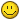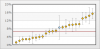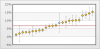## Recommended Posts

When using the ErrorScatter chart, if I have a data point with a y-axis value of 5, and an error value of 6... my lower vertical error bar goes below 0. In many statistical scenarios this does not make any sense. The attribute yAxisMinValue='0' would seem to address this concern, but it appears to be over-ridden by a negative error bar.

Has anyone else had similar problems? Any ideas for how to circumvent it? I have searched the forums and not found it addressed anywhere else. Possibly it is a known issue and is being looked into? Possibly I am an insane person?

If I have not described the problem clearly enough I would be happy to clarify.

Thanks!

p.s. I also thought if I could address the upper and lower error bars separately, I could easily write some code to recalculate the lower value to zero in certain circumstances, but it doesn't look like they are separate elements? Just a thought...

Edited by JimM

##### Share on other sitesHi,

Welcome to FusionCharts Forum!##### Share on other sites

I included 2 screen shots.

The configuration in question would be for the second data point and I am using...

<dataset ...>

.

.

<set x='2' y='2' errorValue='2' />

.

.

</dataset>

In the above case I manually changed the errorValue to 2 because I don't want the bar to go past zero. But the correct errorValue is 5, and the results of this setting can be seen in the second screenshot.

As I mentioned before, this is with <chart ... yAxisMinValue='0' ...>. Is there any other information I can provide? I could clip more of my code or whatever may be helpful. Thanks so much for the attention!

One further point of clarification, in the ideal scenario, the upper error bar would be of length 5, and the lower would be of length 2 (Or whatever length prevented the error bar from going below zero). Thanks again!##### Share on other sites

Well we originally gave up and just used the drawing pad plus annotations to recreate what we wanted, but we'd really like to get back some of the functionality that we sacrificed by doing that. So I'm back to revive this thread. I have included the requested xml below. The data point that is x=65, y=0.5 is the example of the problem in question. The errorValue for this point is 0.87. This means that the max value of the error bar should be 1.37 and the minimum should be -0.37. There are many cases in statistics where reporting an error bar below a value of zero makes no logical sense. As such I would like to represent the errorValue maximum for this point as 1.37, and the minimum as 0.0. Setting the yAxisMin to zero does not seem to have any effect on this. To be honest, I have searched the documentation in depth and am afraid there is simply no way to do this, but we don't have many other options at this point. I can provide any other documentation or answer any questions that you may find helpful. Thank you for your time!

<chart showBorder='0' caption='Rainfall Analysis' yAxisName='Rainfall Rate' xAxisName='Month' verticalErrorBarThickness='1' halfVerticalErrorBar='0' verticalErrorBarColor='5397D9' showvalues='0' bgalpha='0' bgcolor='62B0FF' showalternatehgridcolor='0' canvasborderthickness='1' canvasbordercolor='ffffff,' divlinealpha='10' borderthickness='1' bordercolor='3F3F3F' borderalpha='20' canvasborderalpha='20' outcnvbasefontcolor='3F3F3F' legendbordercolor='DAECFF' legendborderthickness='1' legendBorderThickness ='2' canvasbgcolor='62B0FF,FFFFFF' canvasBgRatio='1,99' canvasBgAngle='90' numberSuffix='%'>

<categories>

<category label='Jan' x='0' />

<category label='Mar' x='20' />

<category label='May' x='40' />

<category label='Jul' x='60' />

<category label='Sep' x='80' />

<category label='Nov' x='100' />

</categories>

<dataset seriesName='2006' color='5397D9' anchorRadius='3' anchorsides='13' anchorBgColor='6BF9E9' anchorBorderThickness='1' >

<set y='11' x='10' errorValue='0.78'/>

<set y='9' x='35' errorValue='0.81'/>

<set y='4' x='50' errorValue='0.68'/>

<set y='14' x='70' errorValue='0.86'/>

<set y='14' x='50' errorValue='0.79'/>

<set y='.5' x='65' errorValue='0.87'/>

<set y='17' x='85' errorValue='0.7'/>

<set y='15' x='15' errorValue='0.82'/>

</dataset>

<dataset seriesName='2007' color='5397D9' anchorRadius='3' anchorsides='13' anchorBgColor='ffffff' anchorBorderThickness='1'>

<set y='14' x='23' errorValue='0.72'/>

<set y='15' x='33' errorValue='0.9'/>

<set y='11' x='77' errorValue='0.83'/>

<set y='13' x='89' errorValue='0.9'/>

<set y='11' x='95' errorValue='0.86'/>

<set y='2' x='4' errorValue='0.7'/>

<set y='7' x='30' errorValue='0.8'/>

<set y='11' x='55' errorValue='0.83'/>

</dataset>

<styles>

<definition>

<style name='myAnchorAnim' type='animation' param='_y' start='0' duration='2'/>

<style name='myErrorAnim' type='animation' param='_yScale' start='0' duration='3' />

</definition>

<application>

<apply toObject='ANCHORS' styles='myAnchorAnim'/>

<apply toObject='ERRORBARS' styles='myErrorAnim'/>

</application>

</styles>

</chart>

Hi,

Could you please send us the relevant XML for the Error Scatter chart so that we might test it?

Edited by JimM

##### Share on other sitesHi,

To show error value above zero, you would need to set x value and errorvalue.

If error value is less than the y-value, then error value will be displayed above 0.( it will be a positive value).

If the error value is greater than the x-value, then the error value will be displayed below 0.(It will be a negative value)

Please note, for displaying error value above 0, you would need to keep x-value greater than the error value.

Ref. Code:

<set y='.87' x='65' errorValue='0.87'/>

Please find attached screen-shot which is rendered using the above configuration.

Hope this helps.

##### Share on other sites

Hi,

Thanks so much for the reply, we have been happy with fusioncharts so far and it is nice to know that your support team is so available!

I gather from your response that you do understand the problem and that there is nothing that can be done about it. Just in the interest of clarification, I would assume that with most statistical charting situations, you do not have the option to choose your x value or your error value at will. These values are set by the data that you are using. The only time where you can reasonably go in and change these values is If your data results in an error value greater than the x value. In this situation you may need to have the error bar below the x value be shorter than the error bar above the x value, so that the error bar does not dip below zero (a situation which makes no statistical sense in many cases.)

I do hope that is clear and understandable. I see that there is not currently any way to do this with the way the errorscatter chart is set up, and that is a fine answer. I just wanted to make known this shortcoming in the event that you run into this problem enough times to necessitate a change.

Thank you!

Jim

P.S. Please let me know if I have in some way misunderstood your answer and there is in fact a way to implement what I am trying to do. Have a good day!

##### Share on other sitesHi,

We have made a note of it, we will pass this information to our developers.

Thanks for the valuable information.